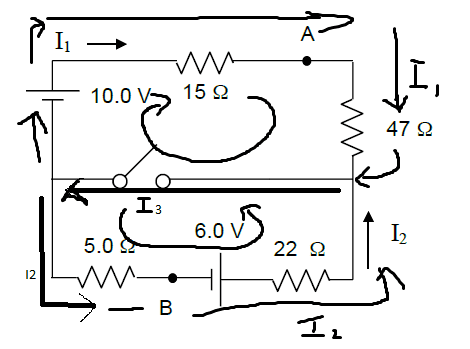# Potential difference between two points (VA - VB)

• johnknee

## Homework Statement

Q. With the switch closed, what is the voltage difference, VA-VB ?## Homework Equations

Junction rule: I3 = I1 + I2
Loop rule(s)

## The Attempt at a Solution

I solved for the currents in this circuit when the switch is closed.

Top loop(clockwise): +10V1 - 15*I1 - 47*I1 + (0V since I'm assuming no Voltage drop across switch) = 0
Bottom loop(counter clockwise): -5*I2 + 6V2 - 22*I2 + 0V = 0

For the top loop: I isolated I1 and got 10/62 = 0.161 = I1
For the bottom loop: I got 6/27 = 0.222A = I2
I1 + I2 = 0.161 + 0.222 = 0.383A.= I3

for the voltage difference VA - VB, I tried doing this with the guidance of a friend.
Va = 10 - I1*15 = 7.585 V.
Vb = Va - Vc - 6 (Vc being the voltage at node C where I3 flows through.)
Then Vc = Va - i1*47 = 7.585 - (7.567) = 0.018 V.
Vb = Va - Vc - 6 = 7.585 - 0.018 - 6 = 1.567 V.
Finally Va - Vb = 7.585 - 1.567 = 6.018 V

I am not sure whether I did this part correct.

You already have a thread dealing with this circuit. You must keep all related questions in that thread.

This thread will be deleted shortly.